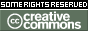REPOZYTORIUM UNIWERSYTETU
W BIAŁYMSTOKUProszę używać tego identyfikatora do cytowań lub wstaw link do tej pozycji: `http://hdl.handle.net/11320/6278`
 Tytuł: Introduction to Stopping Time in Stochastic Finance Theory Autorzy: Jaeger, Peter Słowa kluczowe: stopping timestochastic process Data wydania: 2017 Data dodania: 8-lut-2018 Wydawca: DeGruyter Open Źródło: Formalized Mathematics, Volume 25, Issue 2, Pages 101–105 Abstrakt: SummaryWe start with the definition of stopping time according to , p.283. We prove, that different definitions for stopping time can coincide. We give examples of stopping time using constant-functions or functions defined with the operator max or min (defined in , pp.37–38). Finally we give an example with some given filtration. Stopping time is very important for stochastic finance. A stopping time is the moment, where a certain event occurs (, p.372) and can be used together with stochastic processes (, p.283). Look at the following example: we install a function ST: {1,2,3,4} → {0, 1, 2} ∪ {+∞}, we define:a. ST(1)=1, ST(2)=1, ST(3)=2, ST(4)=2.b. The set {0,1,2} consists of time points: 0=now,1=tomorrow,2=the day after tomorrow. We can prove:c. {w, where w is Element of Ω: ST.w=0}=∅ & {w, where w is Element of Ω: ST.w=1}={1,2} & {w, where w is Element of Ω: ST.w=2}={3,4} andST is a stopping time. We use a function Filt as Filtration of {0,1,2}, Σ where Filt(0)=Ωnow, Filt(1)=Ωfut1 and Filt(2)=Ωfut2. From a., b. and c. we know that:d. {w, where w is Element of Ω: ST.w=0} in Ωnow and{w, where w is Element of Ω: ST.w=1} in Ωfut1 and{w, where w is Element of Ω: ST.w=2} in Ωfut2.The sets in d. are events, which occur at the time points 0(=now), 1(=tomorrow) or 2(=the day after tomorrow), see also , p.371. Suppose we have ST(1)=+∞, then this means that for 1 the corresponding event never occurs.As an interpretation for our installed functions consider the given adapted stochastic process in the article .ST(1)=1 means, that the given element 1 in {1,2,3,4} is stopped in 1 (=tomorrow). That tells us, that we have to look at the value f2(1) which is equal to 80. The same argumentation can be applied for the element 2 in {1,2,3,4}.ST(3)=2 means, that the given element 3 in {1,2,3,4} is stopped in 2 (=the day after tomorrow). That tells us, that we have to look at the value f3(3) which is equal to 100.ST(4)=2 means, that the given element 4 in {1,2,3,4} is stopped in 2 (=the day after tomorrow). That tells us, that we have to look at the value f3(4) which is equal to 120.In the real world, these functions can be used for questions like: when does the share price exceed a certain limit? (see , p.372). Afiliacja: Siegmund-Schacky-Str. 18a, 80993 Munich, Germany URI: http://hdl.handle.net/11320/6278 DOI: 10.1515/forma-2017-0010 ISSN: 1426-2630 e-ISSN: 1898-9934 Typ Dokumentu: Article Występuje w kolekcji(ach): Formalized Mathematics, 2017, Volume 25, Issue 2

Pliki w tej pozycji:
Plik Opis RozmiarFormat
Pozycja ta dostępna jest na podstawie licencji Licencja Creative Commons CCL